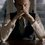# How QM explains Polarization and Magnetization?

I have always got thrilled after reading about Polarization and Magnetization. The former was the first thing I read where QM would have been applied (though I never got a chance to know how). Now, I was learning Magnetization and Magnetic materials. And as I see, it all ends up at the circulating currents - Amperian currents, which "basically" would just be the spin. Spin is an interesting thing to note. As far as I know, Stern-Gerlach experiment was the first evidence of spin. The light split into a finite number of beams. Why? Maybe because that's how the nature is. There is a quantization of angular momentum. It can only take a finite number of values. Nobody knows the basic details.

Now, my question here is how would polarization look like in QM(you may use mathematics of QM, though I know only the basics)? How would it explain it better than CM does?

And how would magnetization look in QM? Dia-, para-, ferro- and all those. Diamagnetism might just be the basic only and can be understood using CM but there is one mean square radius which comes in(or I should say we manipulate it such a way classically so that it becomes same as in QM) the formula of induced diamagnetic moment(for an atom) which seems quite strange in CM(you know the radius of course). Paramagnetism, Ferromagnetism and others are purely quantum mechanical. So, yeah please try to explain them.

It would be nice if an explanation of the "Lande's g factor" is explained before the Magnetization explanation.

Thanks in anticipation!Note by Kartik Sharma
6 years ago

This discussion board is a place to discuss our Daily Challenges and the math and science related to those challenges. Explanations are more than just a solution — they should explain the steps and thinking strategies that you used to obtain the solution. Comments should further the discussion of math and science.

When posting on Brilliant:

• Use the emojis to react to an explanation, whether you're congratulating a job well done , or just really confused .
• Ask specific questions about the challenge or the steps in somebody's explanation. Well-posed questions can add a lot to the discussion, but posting "I don't understand!" doesn't help anyone.
• Try to contribute something new to the discussion, whether it is an extension, generalization or other idea related to the challenge.

MarkdownAppears as
*italics* or _italics_ italics
**bold** or __bold__ bold
- bulleted- list
• bulleted
• list
1. numbered2. list
1. numbered
2. list
Note: you must add a full line of space before and after lists for them to show up correctly
paragraph 1paragraph 2

paragraph 1

paragraph 2

[example link](https://brilliant.org)example link
> This is a quote
This is a quote
    # I indented these lines
# 4 spaces, and now they show
# up as a code block.

print "hello world"
# I indented these lines
# 4 spaces, and now they show
# up as a code block.

print "hello world"
MathAppears as
Remember to wrap math in $$ ... $$ or $ ... $ to ensure proper formatting.
2 \times 3 $2 \times 3$
2^{34} $2^{34}$
a_{i-1} $a_{i-1}$
\frac{2}{3} $\frac{2}{3}$
\sqrt{2} $\sqrt{2}$
\sum_{i=1}^3 $\sum_{i=1}^3$
\sin \theta $\sin \theta$
\boxed{123} $\boxed{123}$

Sort by:

- 6 years ago

Mhmm... a topic for my hot-list. At the moment, I have no clue on the topic. However, soon enough, I will start the "VE Series" (Visual Explanation), where I provide my best explanations of some of the dump-trucked concepts in math & physics. Magnetism is very far on that list, but I'll get there eventually. My first release was pretty popular - the one about the Inverse Square Law - so expect more of these in the future!

But thanks for mentioning me, and sorry I can be of no help for now. Take care!

- 6 years ago